# College Chemistry : Common Ion Effect

## Example Questions

### Example Question #1 : Common Ion Effect

Calculate the molar solubility of barium fluoride when it is dissolved in a solution containing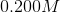of sodium fluoride. Barium fluoride has a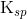value of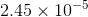.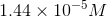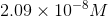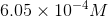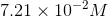Explanation:

Start by writing out the equation for the dissolution of barium fluoride.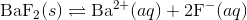Next, write out the chart to keep track of concentrations of each ion.

 [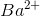] [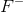] Initial 0.00 0.200 Change +x +2x Equilibrium x 0.200+2x

In the chart,is the amount of barium fluoride that dissolves. Since we are putting barium fluoride in a solution ofsodium fluoride, the initial concentration of the fluoride ion is. Since there is already some fluoride in the solution, we should expect that the molar solubility of barium fluoride should be less than its molar solubility when dissolved in water.

Now, write the equilibrium expression using the chemical equation: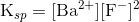Plug in the givenand solve for.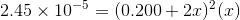At this point, use a graphing calculator to solve for.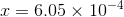The molar solubility of barium fluoride in sodium fluoride is.

### Example Question #2 : Common Ion Effect

Suppose that a chemist wants to lower the concentration of calcium in an aqueous solution by precipitating it out, according to the following reaction expression.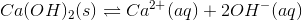Which of the following chemicals could the chemist add to the solution in order to precipitate the calcium?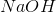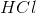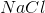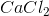Any of theseExplanation:

In this question, we're shown an equilibrium expression for a precipitation reaction in solution. We're asked to identify a compound that will help calcium precipitate out of solution.

To identify a compound that will precipitate calcium out of solution, we need to consider the equilibrium expression shown in the question stem. Applying Le Chatlier's principle, we'll need to consider the direction each of the answer choices will push the reaction in.

Addingwill make the solution more acidic. As a result, there will be a decreased amount of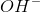. Consequently, the reaction will shift to the right and there will be more calcium in solution.

Addingwill drive up the concentration of calcium, which will drive the reaction to the left. Even though this will cause calcium to precipitate out of the solution, it nonetheless defeats the purpose because the amount of dissolved calcium in solution is not decreasing.

Addingto the solution will have no effect on the reaction equilibrium because neither of the ions appears in the expression.

Addingwill cause the solution to become more basic, with an increased amount of. Consequently, the reaction equilibrium will be driven toward the left. This will, in turn, cause calcium to precipitate out of solution in the form of the solid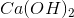.